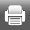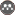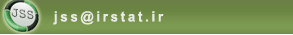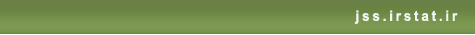[Home ] [Archive]   [ فارسی ]Volume 12, Issue 1 (9-2018)J. of Stat. Sci. 2018, 12(1): 189-208 Back to browse issues page
Spatial Prediction By Using Unilateral Autoregressive Models In Two-Dimensional Space
Abstract:   (2552 Views)

Prediction of spatial variability is one of the most important issues in the analysis of spatial data. So predictions are usually made by assuming that the data follow a spatial model. In General, the spatial models are the spatial autoregressive (SAR), the conditional autoregressive and the moving average models.

In this paper, we estimated parameter of SAR(2,1) model by using maximum likelihood and obtained formulas for predicting in SAR models, including the prediction within the data (interpolation) and outside the data (extrapolation). Finally, we evaluate the prediction methods by using image processing data.

Keywords: Spatial Data, Unilateral Spatial Autoregressive Model, Prediction, Interpolation, Extrapolation
Type of Study: Research | Subject: Spatial Statistics
Received: 2016/05/25 | Accepted: 2017/02/27 | Published: 2018/04/15
Send email to the article author